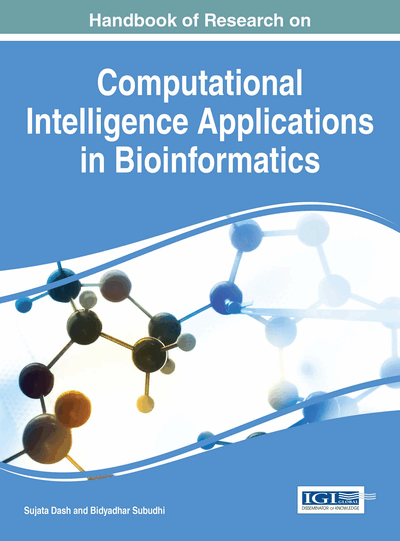# Application of Rough Set Based Models in Medical Diagnosis

Balakrushna Tripathy (VIT University, India)
DOI: 10.4018/978-1-5225-0427-6.ch008
Available
\$37.50
No Current Special Offers

## Abstract

Modeling intelligent system in the field of medical diagnosis is still a challenging work. Intelligent systems in medical diagnosis can be utilized as a supporting tool to the medical practitioner, mainly country like India with vast rural areas and absolute shortage of physicians. Intelligent systems in the field of medical diagnosis can also able to reduce cost and problems for the diagnosis like dynamic perturbations, shortage of physicians, etc. An intelligent system may be considered as an information system that provides answer to queries relating to the information stored in the Knowledge Base (KB), which is a repository of human knowledge. Rough set theory is an efficient model to capture uncertainty in data and the processing of data using rough set techniques is easy and convincing. Rule generation is an inherent component in rough set analysis. So, medical systems which have uncertainty inherent can be handled in a better way using rough sets and its variants. The objective of this chapter is to discuss on several such applications of rough set theory in medical diagnosis.
Chapter Preview
Top

## Definitions And Notations

In this section we provide some definitions and notations to be followed in this chapter. Imprecision in data has become a common feature and hence to handle them imprecise models have been developed in literature. In this sequel we have fuzzy sets, rough sets, intuitionistic fuzzy sets and soft sets along with their generalisations and modifications can be found. Our basic aim in this chapter is to deal with only rough sets. So, we start with the definition of rough sets and related concepts.

Let U be a universe of discourse and R be an equivalence relation over U. By U/R we denote the family of all equivalence classes of R, referred to as categories or concepts of R and the equivalence class of an elementis denoted by [x]R . By a knowledge base, we understand a relational system, where U is as above andis a family of equivalence relations over U. For any subset Q ()P, the intersection of all equivalence relations in Q is denoted by IND(Q) and is called the indiscernibility relation over Q.

## Key Terms in this Chapter

Fuzzy Set: This is yet another imprecise model introduced by L.A. Zadeh in 1965, where the concept of membership function was introduced. Unlike crisp sets here the belongingness of elements to a fuzzy set are graded in the sense that these values can lie in the interval [0, 1].

Decision Table: A decision table is an information table in which the set of attributes is divided into two categories called the condition attributes and the decision attributes.

Rule: A rule is basically an “and…or” rule in the sense that it is an implication where the antecedent part is the conjunction of attributes where aswhereas the consequent part is the disjunction of attributes. They provide some values of the attributes in the antecedent which leads to specific values of the attributes in the consequent part.

Reduct: A reduct is a group of attributes which form a subset of a given set of attributes such that the elements in it cannot be further reduced without losing the representational power of the set. In the rough set terminologyterminology, we say that it is a minimum knowledge under the context.

Core: This is the minimum knowledge in a dataset. In factfact, it is the intersection of all the reducts in a dataset.

Rough Set: It is an imprecise model introduced by Z. Pawlak in 1982, where sets are approximated by two crisp sets with respect to equivalence relations. A set is rough with respect to an equivalence relation or not depending upon whether the lower and upper approximations are not equal or otherwise. Several extensions of this basic model also exist.

Attribute: An attribute defines a characteristic feature of elements in a dataset. These can be numerical or categorical.

## Complete Chapter List

Search this Book:
Reset